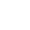# What is place value?You will have learned place value, perhaps without realising. You use it every time you read a number.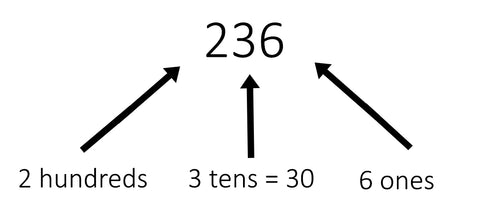A number is written using digits. In 236, the digits are 2, 3 and 6. The place or position of each digit in the number gives it its value.

• In 236 the place value of the ‘2’ is 2 hundreds
• In 623 the place value of the ‘2’ is 2 tens
• In 632 the place value of the ‘2’ is 2 ones
• You may have learned about ‘hundreds, tens and units’ when you were at school. Now teachers often use ‘ones’ instead of units, because ‘unit’ can have other meanings, for example a unit of measurement.

How do children learn place value?

In key stage 1 children begin to read and write two-digit numbers and recognise the place value of each digit.

They represent two-digit numbers with tens and ones blocks:and in place value tables:

 Tens Ones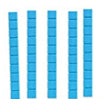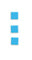5 3

and begin to partition numbers into tens and ones:In key stage 2 they work with larger numbers, starting with hundreds:

 Hundreds Tens Ones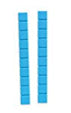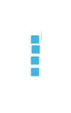1 2 4

and thousands:

 Thousands Hundreds Tens Ones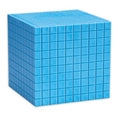1 2 4 4

and then up to millions:

 Millions Hundred Thousands Ten Thousands Thousands Hundreds Tens Ones 4 5 7 2 1 0 8

‘four million, five hundred and seventy-two thousand, one hundred and eight’

Decimal place value

In key stage 2 children also begin to recognise and use place value of decimals , up to three decimal places.

 Ones . tenths hundredths thousandths 3 . 1 0 7

‘three point one zero seven’

An understanding of place value helps your child answer questions like this: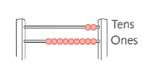• Write the number shown on this abacus
• Write 208 in words
• Write 5.26 in words
• Write three hundred and twenty-one in digits
• Which is larger: 3027 or 3152?
• Both numbers have 3 thousands. Look at the next place value place.

3027 has 0 hundreds

3152 has 1 hundred

So 3152 is larger than 3027

• Make the largest possible number with these digits
•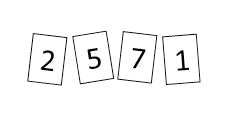Put the largest digit in the highest place value: 7521

• Make the smallest possible number with these digits
•Put the smallest digit in the highest place value: 1257

• What is the value of the 7 in this number: 3720?
• 7 hundred

• Work out the number that is one hundred more than 3419
• Work out the number that is 1000 less than 76210
• Practice at home

Compare numbers in real life contexts – for example which is less: £27 or £35?

Which is the largest football crowd: 5300 or 10200?

Practice spellings of numbers 1-20, the ‘tens’ 30, 40, 50, … and hundred, thousand, etc

To practice place value with your child, Collins Maths Targeted Practice Workbooks are a great tool that target every topic covered in each school year. They include plenty of repeated practice to help your child do their best in Maths.